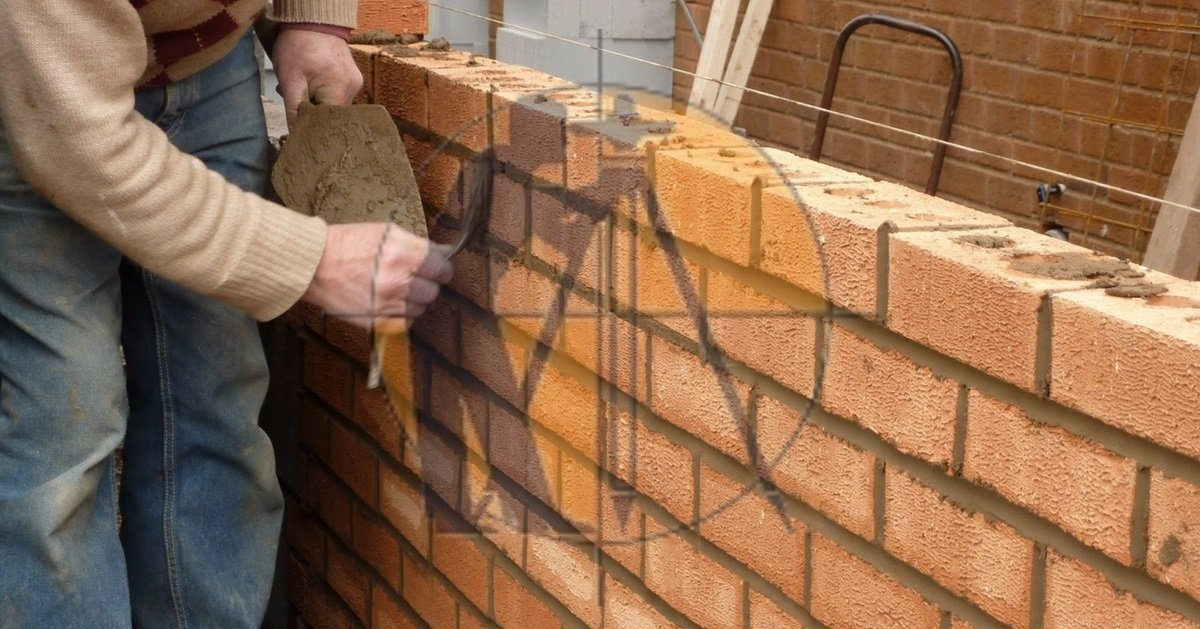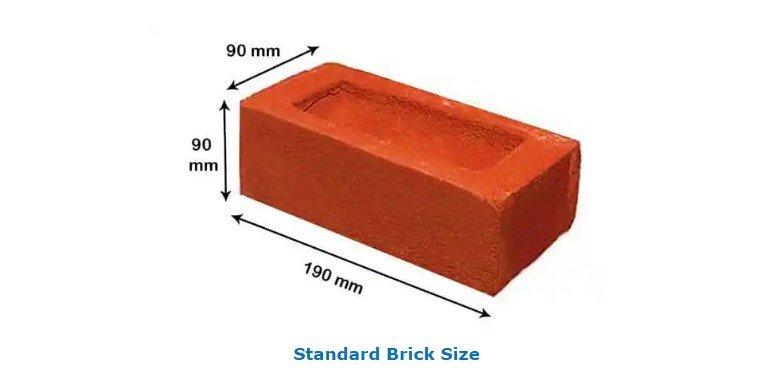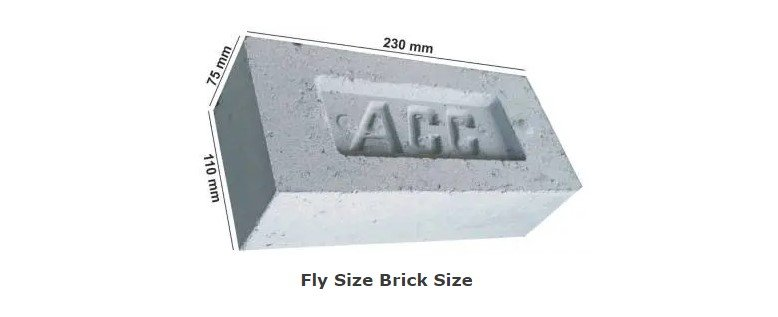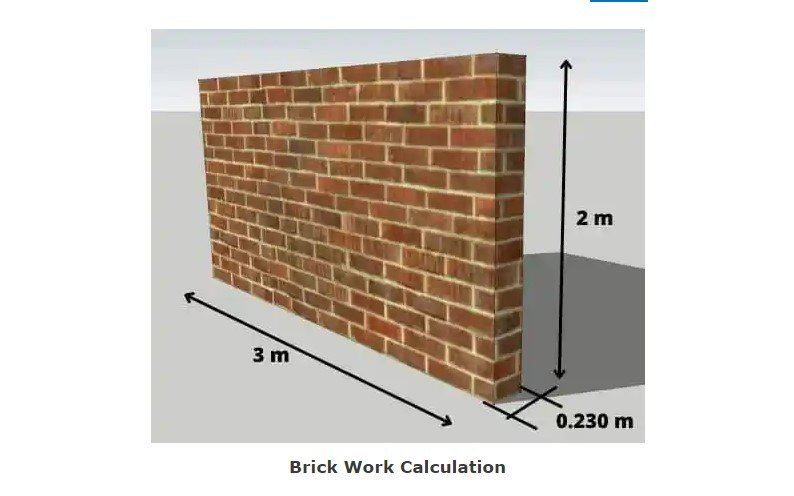Breaking News
Home / Civil Engineering / How to Calculate the Quantity of Cement Mortar In Brickwork and Plaster

# How to Calculate the Quantity of Cement Mortar In Brickwork and Plaster

## What Is Brick work. ?

The Bricks work is the laying of brick in the systematic manner with the help of the mortar in the number of layers. In brick-work, bricks are laid horizontally side by side & 1 above the other to make them strong. The structure of formed by placing brick in such a manner is called a brick masonry wall.

Brick work calculations & how to calculated QTY of cement mortar in brickwork & plaster is given below.## How Many Types of Bricks used in Construction?

The three most used bricks used in Building Construction are as follows.

## Standard Size of Brick

### 1 . Red BricksThe standard Size of the Brick in mm is 190 x 90 x 90 mm ( Length x Width x Thickness)

## Civil Engineering Technology

### Bar Bending Schedule For Reinforcement Beam

The Standard Brick Dimensions Inches is 7.48 In X 3.54 In X 3.54 In (Length x Width x Thickness)

### 2 . Fly Ash BrickThe standard size of the fly ash brick is 230-mm x 110-mm x 75-mm used in Construction.

### 3 . Hollow Brick

The standard size of the hollow brick is 30 x 15 x 15cm.

## How to Calculate the Number of Brick in a wall

First of all, we do a brick work calculation for 1-m3 of brickwork volume.

The volume of the brick work = 1-m3

Size of the brick with mortar = 20cm x 10cm x 10cm

The Standard size of the brick without mortar = 19cm x 9cm x 9cm.

Nos of the brick required in 1-m3 = 1/(0.20×0.10×0.10) = 500Nos.

### Bar Bending Schedule For Circular Column

500 is the number of the bricks required in 1-m3 with mortar. It means mortar volume is also calculated in the calculations. So we have to find out the actual numbers of bricks & the QTY of mortar.

Actual volume of the Brick = 500 x (0.19 × 0.09 × 0.09) = 0.7695 m3

Volume of the Mortar = 1 – 0.7695 = 0.2305 m3

Adding ten%  wastage  = 0.2305 + (0.2305×10/100) = 0.2305 + 0.02305 = 0.25355 m3

As this is the wet volume of the mortar, the dry volume is generally twenty five% more than wet volume.

Add twenty five% Extra volume for the dry volume of mortar.

= 0.25355 + (0.2355 × 25 / 100) = 0.25355 + 0.0633875 = 0.3169 m3

#### So, from the above calculation we can say that

Nos. of Brick required for one m3 of brick work = 500

Mortar required for one m3 of brick = 0.3169 m3

## How to Calculate the QTY of Cement Mortar in Brickwork & Plaster

### 1 . QTY of the Cement Mortar In Brick work CalculationLet’s Take an example, The length of wall = three mwidth = two m, & thickness of the wall = 0.23-m.

Volume of the Brickwork = 9 x 5 x 0.23 = 10.35-m3

We know that, for one m3 brickwork = 500 Nos required

So, for 10.35-m3 of the brick work = 500x 10.35 = 5175 Nos.

Now Also , know that for one m3 of the brickwork 0.3169 m3 of mortar is required

So, for 10.35 m3 of the brickwork = 0.3169 × 10.35 = 3.28 m3

Now, Let us find out the QTY of cement & sand in mortar,

### Mix Ratio 1:4

#### Consider mortar mix ration is 1:4

one part = cement, four part = sand

Total part = 1 + 4 = 5 part

### 1 . Cement ( bags )

Cement = 1 / 5  x (volume of the mortar)

= 1 / 5  x  3.28

= 0.656 m3 ( volume of one bag of cement = 0.035 m3 )

= 0.656 / 0.035

Cement = 18.74 bags = 19 bags

### 2 . Sand ( cu-ft. )

Sand = 4 / 5 x 3.28

Sand = 2.62 m3

### For Mix Ratio ( 1:6 )

Cement = one part, Sand = Six part

Total Part = 1 + 6 = 7 part

### 1. Cement ( Bags )

Cement = 1/7 x 3.28

Cement = 0.46 m3 ( One bag volume of the cement = 0.035 m3 )

Cement = 13.14 bags

### 2. Sand ( cu- ft. )

Sand = 6/7 x 3.28

Sand = 2.18 m3

### Summary.

#### 1 . For Cement Mortar (1:4)

Cement = 19 bags , Sand = 2.62 m3 ( 92.52 ft3 )

#### 2 . For Cement Mortar (1:6)

Cement = 13 bags, Sand = 2.18 m3 ( 77 ft3 )

## Cement Mortar Calculation In The Plasterwork.

Consider a wall having length = fifteen mwidth = Eight m with plasterwork thickness = 12 mm (0.012m)

First, Calculate The Volume of Cement Plaster work

Volume of  the Cement Mortar in Plastering = 15 x 8 x 0.012 = 1.44 m3

Adding Ten% wastage = 1.44 + 1.44 x (10 / 100) = 1.44 + 0.144 = 1.584 m3 (wet volume of the mortar)

As this is wet volume of the mortar, the dry volume is generally Twenty five% more than wet volume.

Add Twenty Five % Extra volume for the dry volume of mortar.

= 1.584 + ( 1.584 × 25 / 100 ) = 1.584 + 0.1584 = 1.74 m3

### Cement Mortar Mix Ratio = ( 1:4 )

One part cement & Four part sand ( Total Part = ( 1 + 4 ) = 5 part

### 1. Cement ( One part )

Cement = ( 1 / 4 ) x 1.74

= 0.435 m3 (One bag volume of the cement = 0.035 m3)

= 0.435 / 0.035 = 12.42

### 2 . Sand ( Four part )

Sand = 4 / 5 x 1.74

Sand = 1.392 m3

### Cement Mortar Mix Ration = ( 1:6 )

Cement part = One  , Sand Part = Six

Cement = ( 1 / 7 ) x 1.74

= 0.24 m3  ( One bag volume of the cement = 0.035 m3 )

= 0.24 / 0.035 = 7.10

### 2 . Sand ( Four part )

Sand = 6 / 7 x 1.74

Sand = 1.49 m3

### Summary.

For ( 1:4 ) Mix Cement Mortar : Cement = 12.42 bags, Sand 1.392 m3 ( 49.15 ft3 )

For ( 1:4 ) Mix Cement Mortar : Cement = 7.10 bags, Sand 1.49 m3 ( 52.61 ft3 )

## Other Post

### THANKS.